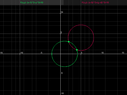# 3.1.12 intersecting circles

• MHB
Gold Member
MHB
Find the coordinates of all points
whose distance form (1,0) is $\sqrt{10}$
and whose distance is from (5.4) is $\sqrt{10}$

ok assume first we convert the info to (2) general eq of a circle
$(x-h)^2+(y-k)^2=r^2$
so we have
$(x-1)^2+(y-0)^2=10$ and $(x-4)^2+(y-4)^2=10$

edit:took out tikz

Last edited:

skeeter
ok assume first we convert the info to (2) general eq of a circle
$(x−h)^2+(y−k)^2=r^2$
so we have
$(x−1)^2+(y−0)^2=10$ and $(x−{\color{red}5})^2+(y−4)^2=10$

fixed

Gold Member
MHB
Find the coordinates of all points
whose distance form (1,0) is $\sqrt{10}$
and whose distance is from (5.4) is $\sqrt{10}$

$(x-h)^2+(y-k)^2=r^2$
so we have
$(x-1)^2+(y-0)^2=10$ and $(x-5)^2+(y-4)^2=10$
these eq are equal to each other so expand and combine like terms
$(x-1)^2+(y-0)^2=(x-5)^2+(y-4)^2$
$x^2-2x+1+y^2=x^2-10x+25+y^2-8y+16$
$-2x+1=-10x-8y+41$
$8y=-8x+40$
$y=-x+5$
so far?
W|A says but ?
x = 2, y = 3
x = 4, y = 1

W|A

edit: took out tikz

Last edited:
skeeter
$y = 5-x \implies (x-1)^2 + (5-x)^2 = 10$

solve for the two values of x, then the two corresponding values of y

Last edited by a moderator:
Gold Member
MHB
$(x-1)^2 + (5-x)^2 = 10$
$x^2-2x+1+25-10x+x^2=10$
$2x^2-12x+16=0$
$x^2-6x+8=0$
$(x-2)(x-4)=0$
$x=2\quad x=4$
$y=5-2=3$
$y=5-4=1$
hence pts of intersection are (2,3),(4,1)
tikz isn't correct

edot: fixed tikz cirlcles

\begin{tikzpicture}[xscale=.3,yscale=.3]
\draw [thin] (0,-5) -- (0,10);
\draw [thin] (-5,0) -- (10,0);
\draw[thick] (1,0) circle (3.162277);
\draw[thick] (5,4) circle (3.162277);
\draw[thin][fill] (2,3) circle (.2);
\draw[thin][fill] (4,1) circle (.2);
\end{tikzpicture}

Last edited:
skeeteryep … what did you do wrong?

Gold Member
MHB
the radius had to be $\sqrt{10}$ not 10
but now need to put ticks and text for intersection pts (2,3)(4,1)

nice graph but I like more control of ticks

Last edited: